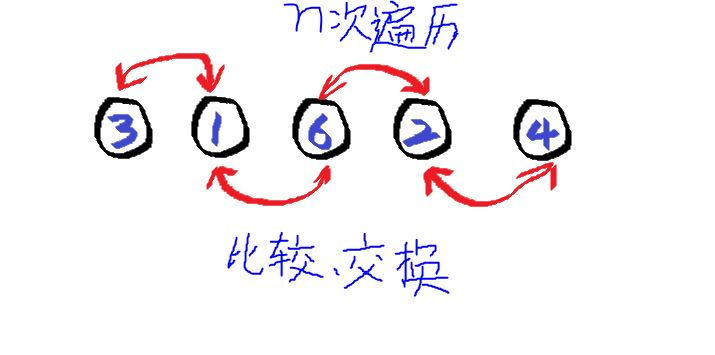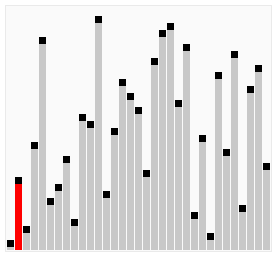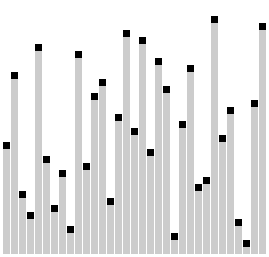# 排序2-冒泡排序

## 经典冒泡排序int classic_bubble_sort(int *sort_butt, int size, bool ascend)
{
int i = 0, idx = 0;
int end_idx = size-1;
int swap_tmp = 0;

for (i = 0; i < size-1; i++) {
for (idx = 0; idx < end_idx; idx++) {
if (sort_butt[idx] > sort_butt[idx+1]) {
swap_tmp = sort_butt[idx];
sort_butt[idx] = sort_butt[idx+1];
sort_butt[idx+1] = swap_tmp;
}
}
}

nb_logd("[%s] ", __FUNCTION__);
return 0;
}


1. 利用标志位（类似哨兵）来标识某一次循环是否有交换操作，如果没有则就此结束排序，因为此时数组就已经是有序的了。
2. 内层循环每次循环之后就减去1个循环长度，因为每次循环过后就把最大（最小）的那个数放在了数组末尾，下次就无需再次全部遍历了。

int fast_bubble_sort(int *sort_butt, int size, bool ascend)
{
int i = 0, idx = 0;
int end_idx = size-1;
int swap_tmp = 0;
bool swap_flag = 0;

for (i = 0; i < size-1; i++) {
for (idx = 0; idx < end_idx; idx++) {
if (sort_butt[idx] > sort_butt[idx+1]) {
swap_tmp = sort_butt[idx];
sort_butt[idx] = sort_butt[idx+1];
sort_butt[idx+1] = swap_tmp;
swap_flag = 1;
}
}
if (!swap_flag)
goto end;

swap_flag = 0;
end_idx--;
}

end:
nb_logd("[%s] ", __FUNCTION__);
return 0;
}


## 鸡尾酒排序

int shaker_bubble_sort(int *sort_butt, int size, bool ascend)
{
int i = 0, idx = 0;
int end_idx = size-1, start_idx = 0;
int swap_tmp = 0;
bool swap_flag = 0;

while (start_idx < end_idx) {
for (idx = start_idx; idx < end_idx; idx++) {
if (sort_butt[idx] > sort_butt[idx+1]) {
swap_tmp = sort_butt[idx];
sort_butt[idx] = sort_butt[idx+1];
sort_butt[idx+1] = swap_tmp;
swap_flag = 1;
}
}
if (!swap_flag)
goto end;
swap_flag = 0;
end_idx--;

for (idx = end_idx; idx > start_idx; idx--) {
if (sort_butt[idx] < sort_butt[idx-1]) {
swap_tmp = sort_butt[idx];
sort_butt[idx] = sort_butt[idx-1];
sort_butt[idx-1] = swap_tmp;
swap_flag = 1;
}
}

if (!swap_flag)
goto end;
swap_flag = 0;
start_idx++;
}

end:
nb_logd("[%s] ", __FUNCTION__);
return 0;
}## 梳排序int gap_bubble_sort(int *sort_butt, int size, bool ascend)
{
int i = 0, idx = 0;
int end_idx = 0;
int swap_tmp = 0;
int gap = size;
bool swap_flag = 1;

while(swap_flag || gap != 1) {
swap_flag = 0;
gap /= 1.3;
gap = (gap < 1) ? 1 : gap;

end_idx = size-gap;
for (idx = 0; idx < end_idx; idx++) {
if (sort_butt[idx] > sort_butt[idx+gap]) {
swap_tmp = sort_butt[idx];
sort_butt[idx] = sort_butt[idx+gap];
sort_butt[idx+gap] = swap_tmp;
swap_flag = 1;
}
}
}

end:
nb_logd("[%s] ", __FUNCTION__);
return 0;
}


Gap比例 数组长度 外层循环次数
1.3 5000 33
1.5 5000 531
1.2 5000 41

## End06-1423万+

#### 冒泡排序的2种写法

09-24159万+

#### 经典算法---冒泡排序09-20203

#### 排序算法（2）冒泡排序法

02-228398

#### 改进优化冒泡排序

05-13155

#### 排序算法2：冒泡排序

04-14298

#### 排序5-线性排序

06-057425

#### 冒泡排序的优化及最好最差情况

07-053841

#### 冒泡排序

03-2484

#### 数据结构排序算法2-冒泡排序

03-151556

#### Java 排序算法-冒泡排序及其优化

11-231679

#### 带flag标准位的冒泡排序

07-28891

#### Java数组排序-冒泡排序

02-252663

#### Java学习笔记排序算法-------冒泡排序

04-28930

#### java排序--交换排序--冒泡排序和快速排序

05-0363

#### 2、冒泡排序（详细）

11-17926

#### Python四大流行排序算法详解--快速排序-冒泡排序-选择排序-插入排序。

11-26298

#### 冒泡排序出现错误

09-26109

#### 冒泡排序 --分析及代码实现（含改进）©️2020 CSDN 皮肤主题: 大白 设计师: CSDN官方博客点击重新获取扫码支付1.余额是钱包充值的虚拟货币，按照1:1的比例进行支付金额的抵扣。
2.余额无法直接购买下载，可以购买VIP、C币套餐、付费专栏及课程。余额充值